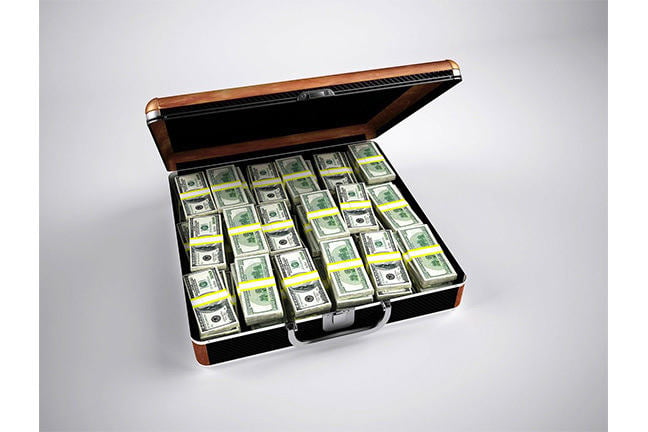3.13

## UNSW SydneyMoney

# Pareto and the distribution of wealth

More than a hundred years ago, the Italian economist Vilfredo Pareto (1848- 1923) studied income and wealth distributions. And he noted a curious fact: that certain power laws seemed to crop up in many different contexts to explain distributions of wealth.

In this step we look at how Pareto distributions follow a power law, but this time with a negative exponent, and how these shed light on wealth distributions in the modern world.

## The 80-20 rule

There is an old rule in economics, that the top $\normalsize{20\%}$ own $\normalsize{80\%}$ of everything. And of that top $\normalsize{20\%}$, the top $\normalsize{20\%}$ of them own $\normalsize{80\%}$ of the $\normalsize{80\%}$. And so on. Economists refer to such a distribution as scale-free.

Q1 (E): What percentage does the top $\normalsize 20\%$ of the top $20\%$ represent? How much would they own by the $\normalsize 80$-$\normalsize 20$ rule?

This kind of rule of course needs to be taken with a bit of latitude. It is not always rigidly $\normalsize{80/20}$. Over time a given society may become more “top heavy” and then less so. For example in the US, the 1920’s were a time of considerable income disparity, but then that reduced gradually, and then started to increase again in the 1970’s, and is now roughly back at similar levels to the 1920s.

Nevertheless, it seems generally to apply to quite a lot of things. In stores, $\normalsize{20\%}$ of customers account for $\normalsize{80\%}$ of sales. On the internet, $\normalsize{20\%}$ of sites get around $\normalsize{80\%}$ of the traffic.

## Pareto’s observation

Pareto realized that behind the $\normalsize{80/20}$ rule was a particular distribution: in fact a power law, but now with a negative exponent.Let’s consider a general Pareto-type curve of the form

for some exponent $\normalsize{\alpha}$ usually greater than $\normalsize{1}$. The case of $\normalsize{\alpha=1}$ is rather a special case due to the unique properties of the inverse relation $\normalsize{y=1/x}$.

Now how does these abstract mathematical curves relate to real-world economics? Pareto proposed that the number $\normalsize N$ of people that had an income bigger than $\normalsize x$ satisfied a Pareto type distribution:

for some constants $\normalsize A$ and $\normalsize \alpha$.

## The $\normalsize 1 \%$ rule of internet content creation

Other manifestations of this kind of phenomenon abound. For example, a related internet rule of thumb is that only $\normalsize 1 \%$ of internet users on a channel will create content. A variant of this is the 90–9–1 version of the rule, which states that for websites where users can both create and edit content, 1% of people create content, 9% edit or modify that content, and 90% view the content without contributing.

## Discussion

What do you think about the relevance of such rough laws for FutureLearn courses? And perhaps more delicate, but also more interesting: how does income distribution work in your country? Is it an 80 /20 rule? Or something else?

A1. The top $\normalsize 20 \%$ of the top $\normalsize 20 \%$ is the top $\normalsize 4 \%$ of everyone. They will, assuming the 80-20 rule, own $\normalsize 80 \%$ of $\normalsize 80 \%$ of everything, which is $\normalsize 64 \%$ of everything. Who said life was fair?